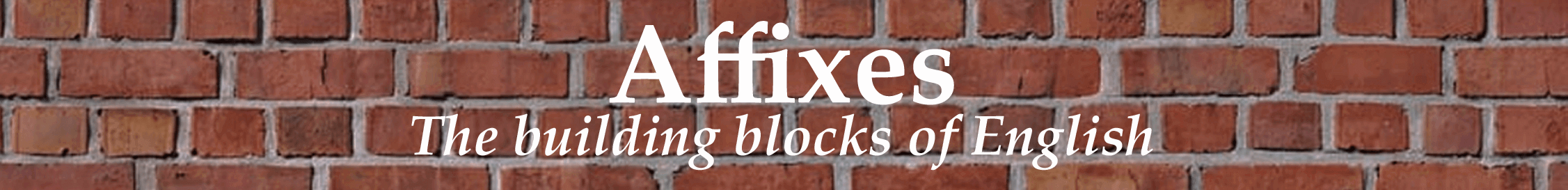# deci-

One tenth.

Latin decimus, tenth.

The decimal system of counting is one of numbers and arithmetic based on the number ten; to decimate originally meant to kill one in every ten of a group, but in current usage it refers to any large proportion; a decile is each of ten equal groups into which a population can be divided according to the distribution of values of a variable. For decillion see the entry <Number words. A decibel (one tenth of a bel, a unit too large for practical convenience) is used to measure the intensity of a sound or an electrical signal by comparing it with a given level on a logarithmic scale; other examples in units of measurement include decilitre and decimetre (US deciliter and decimeter). It is one of the standard SI (Système International) multiples (see the entry Number words).

Visit Michael Quinion’s World Wide Words site for 3000+ articles on English!